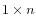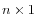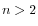The free energy minimization algorithm includes a number of advanced options:
• Avoid isolated base pairs. The algorithm filters out isolated base pairs (i.e. stems of length 1).
• Apply different energy rules for Grossly Asymmetric Interior Loops (GAIL). Compute the minimum free energy applying different rules for Grossly Asymmetry Interior Loops (GAIL). A Grossly Asymmetry Interior Loop (GAIL) is an interior loop that isorwhere(see http://www.bioinfo.rpi.edu/~zukerm/lectures/RNAfold-html/rnafold-print.pdf).
• Include coaxial stacking energy rules. Include free energy increments of coaxial stacking for adjacent helices [Mathews et al., 2004].
• Apply base pairing constraints. With base pairing constraints, you can easily add experimental constraints to your folding algorithm. When you are computing suboptimal structures, it is not possible to apply base pair constraints. The possible base pairing constraints are:
• Force two equal length intervals to form a stem.
• Prohibit two equal length intervals to form a stem.
• Prohibit all nucleotides in a selected region to be a part of a base pair.
Base pairing constraints have to be added to the sequence before you can use this option - see below.
• Maximum distance between paired bases. Forces the algorithms to only consider RNA structures of a given upper length by setting a maximum distance between the base pair that opens a structure.

Subsections